NumPy Arrays, are arrays, build in Python with all the basic functionalities which an array has, such as split, reshape, join. etc.

Many Python packages for Numerical/Scientific computing such as pandas are built around NumPy and use its array feature to build their functions.

Let us begin by creating a simple 1-dimensional array in NumPy.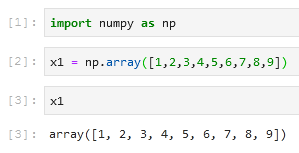Not just 1-dimensional, but we can create higher-dimensional arrays too.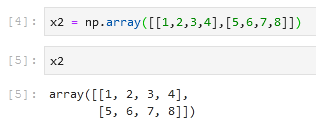### Array Attributes in NumPy

#### Number of dimensions

This attribute tells us the number of dimensions of the array.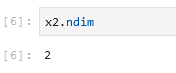#### The Shape of the Array

This gives us the number of rows and columns in the array.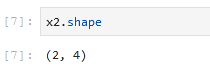#### Size of the Array

This gives us the total size (number of items) of the array.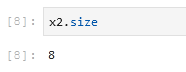#### Data Type of the Array

This gives us the data type of the items in the array.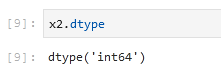#### Size of each item in Array

This gives us the size of each item in bytes in the array.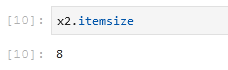#### Size (in bytes) of the Array

This gives us the size of the complete array in bytes.